# Solution assignment 06 Fractional functions and graphs

### Assignment 6

Given the function: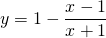Find:
the vertical asymptote;
the horizontal asymptote;
the intersection point with the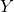-as if it exists;
the intersection point with the-as if it exists.

Based on these results sketch the result in the figure.

### Solution

This function looks slightly different than we are used to, but after a few calculations we come on familiar ground.
We rewrite: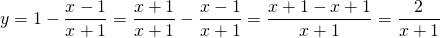Now this function has the familiar form.
The vertical asymptote of this function is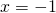, i.e. the value for which the denominator equals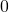. We find the horizontal asymptote by trying to find the value of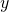if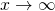orand that is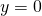, thus the-axis. Furthermore we notice that the intersection point with the-axis (take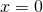) is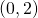. There is no intersection point with the-axis because the-axis is an asymptote.
The graph is depicted in the following figure.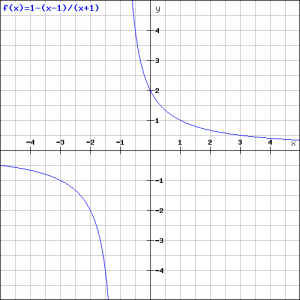0